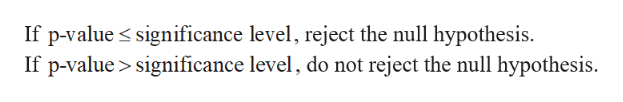Question
1 views
check_circle

Step 1

(a)

The conditions for the t-distribution is appropriate as given below:

• If the sample size is larger (n≥30) or the distribution follows normal, then the t-distribution is appropriate.
• If the sample size is small and heavy skewness with outliers, then the t-distribution is not appropriate.

Here, the sample size is larger (n(=65)≥30). Hence, the condition for normality is satisfied. Thus, the t-distribution is appropriate.

(b)

The hypotheses are given below:

Null hypothesis:

H0:μ=\$43,900

Alternative hypothesis:

H1:μ≠\$43,900

(c)

The test statistic is obtained as follows:

Step 2

Thus, the test statistic is 0.383.

(d)

The degrees of freedom is obtained as follows:

p-value:

Step-by-step procedure to obtain the p-value using StatKey:

• Choose t under Theoretical Distributions.
• In degrees of freedom enter 64 and click Ok.
• Click Two Tail.
• In Custom cutoff, enter the numerical cutoff value as –4.45.
• Click OK.

Output obtained using StatKey is as follows:

Step 3

From the output, the p-value is 0.702 (=0.351*2).

(e)

...help_outlineImage TranscriptioncloseIf p-value significance level, reject the null hypothesis If p-value> significance level, do not reject the null hypothesis. fullscreen

### Want to see the full answer?

See Solution

#### Want to see this answer and more?

Solutions are written by subject experts who are available 24/7. Questions are typically answered within 1 hour.*

See Solution
*Response times may vary by subject and question.
Tagged in
MathStatistics

### Hypothesis Testing